Search datasheet (1.687.043 components) Search fieldPart namePart descriptionAbout site Manufacturers list ChipFindIC search engine AllXrefCross-reference database### Datasheet: SDW80S600 (SemiWell Semiconductor)

Fast Soft Recovery Rectifier Diode
Download:PDFZIP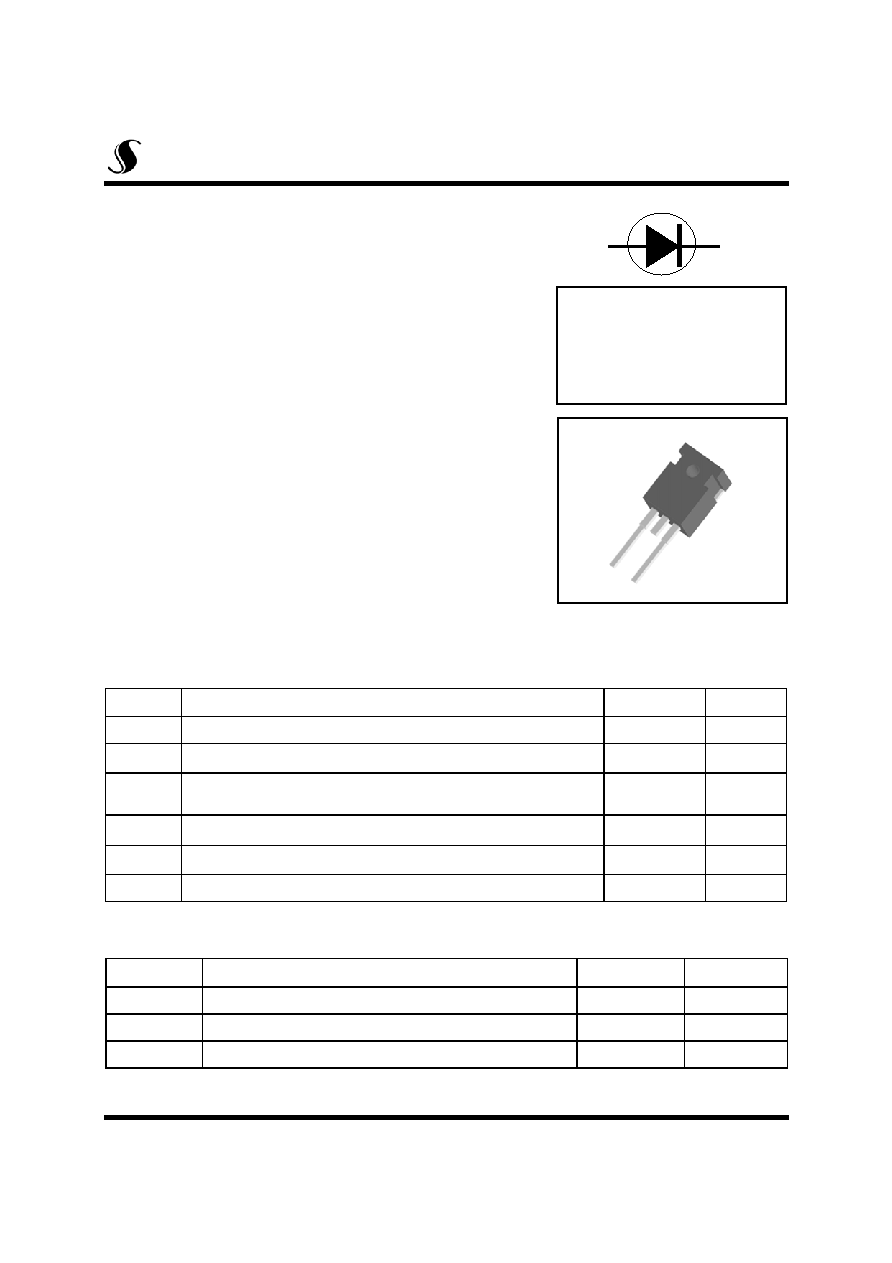1
2
1.Cathode 2. Anode
Symbol
Parameter
Value
Units
V
RRM
Repetitive Peak Reverse Voltage
600
V
I
F(AV)
Average Rectified Forward Current @ T
C
= 82�C
80
A
I
FSM
Non-Repetitive Peak Surge Current (t=8.3ms)
(Surge applied at rated load conditions half sinewave,single phase, 60HZ)
800
A
I
2
t
I
2
t for Fusing ( t = 8.3ms ,60Hz sine pulse )
2650
A
P
tot
Total Power Dissipation @ T
C
= 25�C
270
W
T
J/
T
STG
Maximum Junction / Storage Temperature Range
- 40 ~ 150
�C
Symbol
Parameter
Value
Units
R
thJC
Maximum Thermal Resistance, Junction-to-Case
0.47
�C/W
R
thCS
Typical Thermal Resistance, Case-to-Heatsink
0.25
�C/W
R
thJA
Maximum Thermal Resistance, Junction-to-Ambient
35
�C/W
SDW80S600
SemiWell
Semiconductor
Fast Soft Recovery Rectifier Diode
SDW80S600
SDW80S600
March, 2003. Rev. 01
Features
Plastic material meets UL94V-0
Glass passivated chips
Very short recovery time
Extremely low switching loss
Soft recovery behavior
High ruggedness type
General Description
The SDW80S600 FRD has been designed for applications
requiring low forward voltage drop and soft recovery charac-
teristics. Typical applications are output rectification for
SMPS, UPS, Welding and freewheeling in inverter, chopper
and converter.
I
FAVM
= 80A
V
RRM
= 600V
t
rr
= 65ns
1/5
Absolute Maximum Ratings
Thermal Characteristics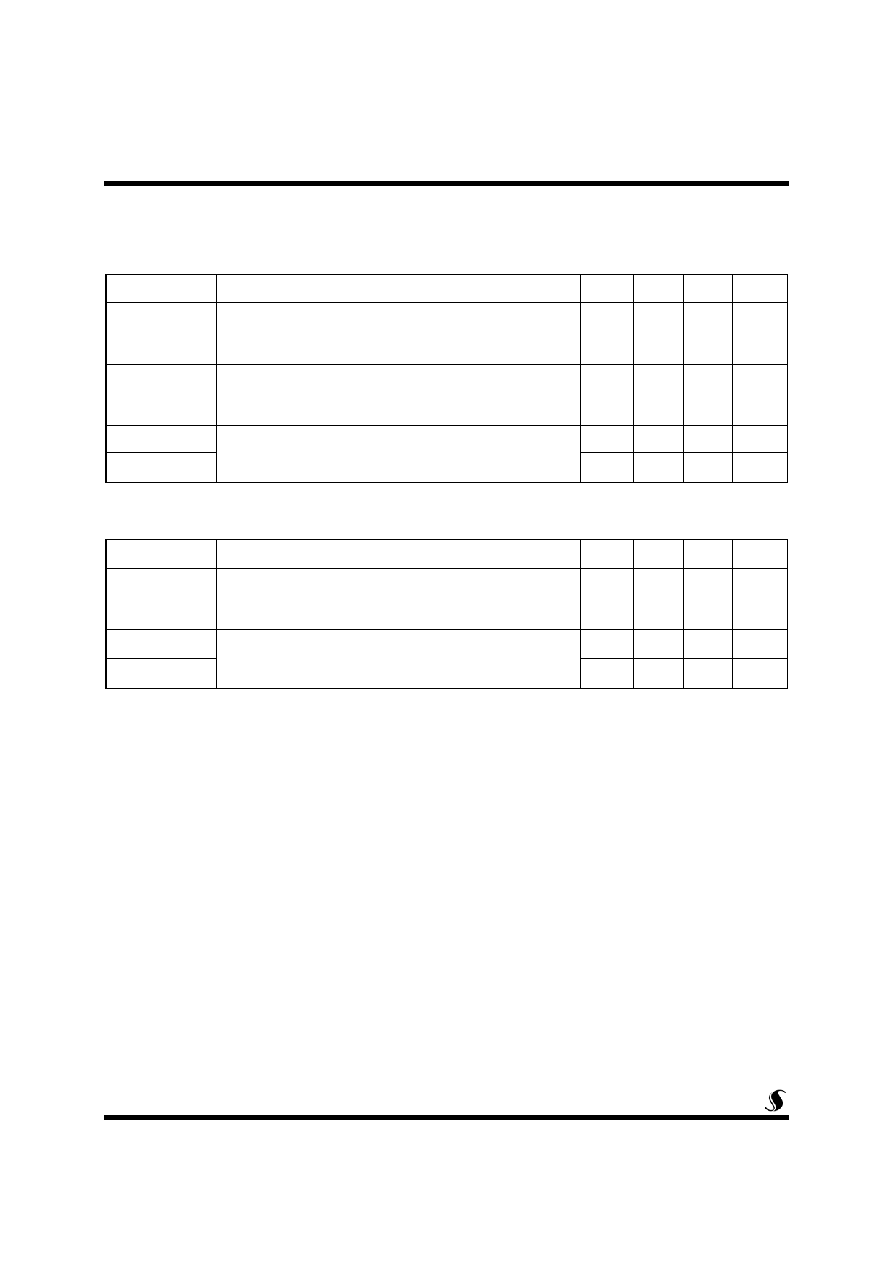Electrical Characteristics
Symbol
Parameter
Min
Typ
Max
Units
I
R
Reverse Leakage Current
V
R
= V
RRM
T
C
= 25 �C
T
C
= 150 �C
-
-
-
-
0.1
14
mA
V
F
Forward Voltage Drop
I
F
= 80A T
C
= 25 �C
I
F
= 80 A T
C
= 150 �C
-
-
1.5
1.3
1.8
1.5
V
rt
Forward Slope Resistance, Tj =150�C
Threshold Voltage
-
-
8.0
m
V
F
(TO)
-
-
0.85
V
Symbol
Parameter
Min
Typ
Max
Units
t
rr
Reverse Recovery Time
I
F
= 40A , V
R
=30V ,di/dt = -50A/us
I
F
= 1A , V
R
=30V ,di/dt = -50A/us
-
-
80
65
130
100
ns
I
rr
Reverse Recovery Current
I
F
= 40A , V
R
=30V ,di/dt = -50A/us
Reverse Recovery Charge
-
-
3.5
A
Q
rr
-
-
0.5
uC
SDW80S600
2/5
Recovery Characteristics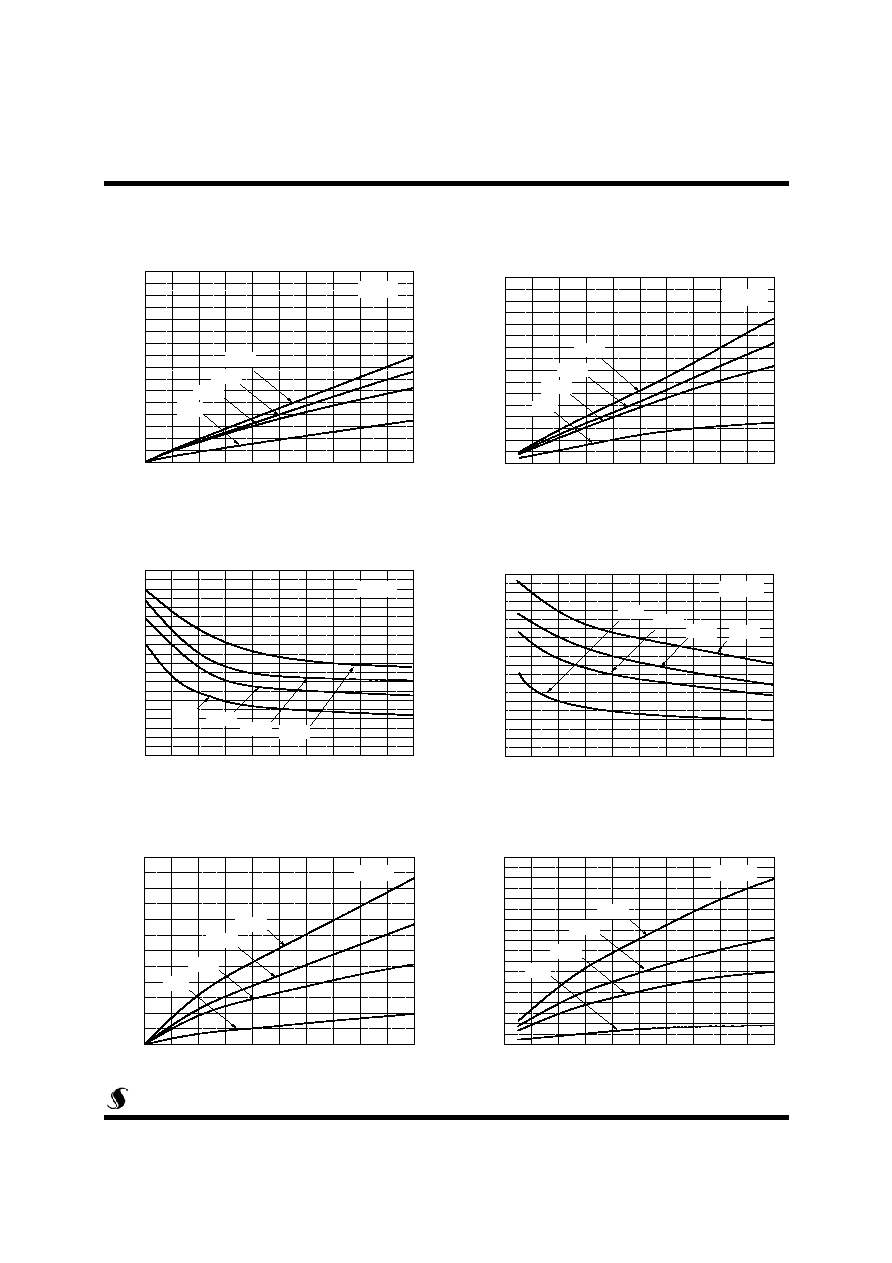0
40
80
120
160
200
0
5
10
15
20
25
30
35
40
T
C
=150
o
C
I
RM
, Reve
rse recovery
current[A
]
di/dt, Rating of decreasing forward current[A/us]
I
F
=40A
I
F
=20A
I
F
=10A
I
F
=1A
0
40
80
120
160
200
0
40
80
120
160
200
240
280
320
360
400
T
C
=150
o
C
Trr, R
e
verse r
e
covery
t
i
me[
n
s]
di/dt, Rating of decreasing forward current[A/us]
I
F
=40A
I
F
=20A
I
F
=10A
I
F
=1A
0
40
80
120
160
200
0
5
10
15
20
I
F
=40A
I
F
=20A
I
F
=10A
I
F
=1A
I
RM
,
R
e
ve
rse reco
very cu
rre
n
t[
A
]
di/dt, Rating of decreasing forward current[A/us]
T
C
=25
o
C
0
40
80
120
160
200
0
100
200
300
400
500
600
T
C
=25
o
C
Q
rr
,
Re
ve
rs
e r
e
co
ve
r
y
c
h
a
r
g
e
[
n
C]
di/dt, Rating of decreasing forward current[A/us]
I
F
=40A
I
F
=20A
I
F
=10A
I
F
=1A
0
40
80
120
160
200
0
400
800
1200
1600
2000
2400
2800
3200
3600
T
C
=150
o
C
Q
rr
,
Re
ve
rse
re
co
ve
ry
ch
arg
e
[
n
C
]
di/dt, Rating of decreasing forward current[A/us]
I
F
=40A
I
F
=20A
I
F
=10A
I
F
=1A
0
40
80
120
160
200
0
20
40
60
80
100
120
140
160
180
200
T
C
=25
o
C
T
C
=25
o
C
T
rr, Re
verse re
covery
time[ns]
di/dt, Rating of decreasing forward current[A/us]
I
F
=40A
I
F
=20A
I
F
=10A
I
F
=1A
3/5
Fig 3. di/dt vs. reverse recovery time
(T
C
=25
o
C)
Fig 4. di/dt vs. reverse recovery time
(T
C
=150
o
C)
SDW80S600
Fig 1. di/dt vs. maximum reverse current
(T
C
=25
o
C)
Fig 2. di/dt vs. maximum reverse current
(T
C
=150
o
C)
Fig 5. di/dt vs. reverse recovery charge
(T
C
=25
o
C)
Fig 6. di/dt vs. reverse recovery charge
(T
C
=150
o
C)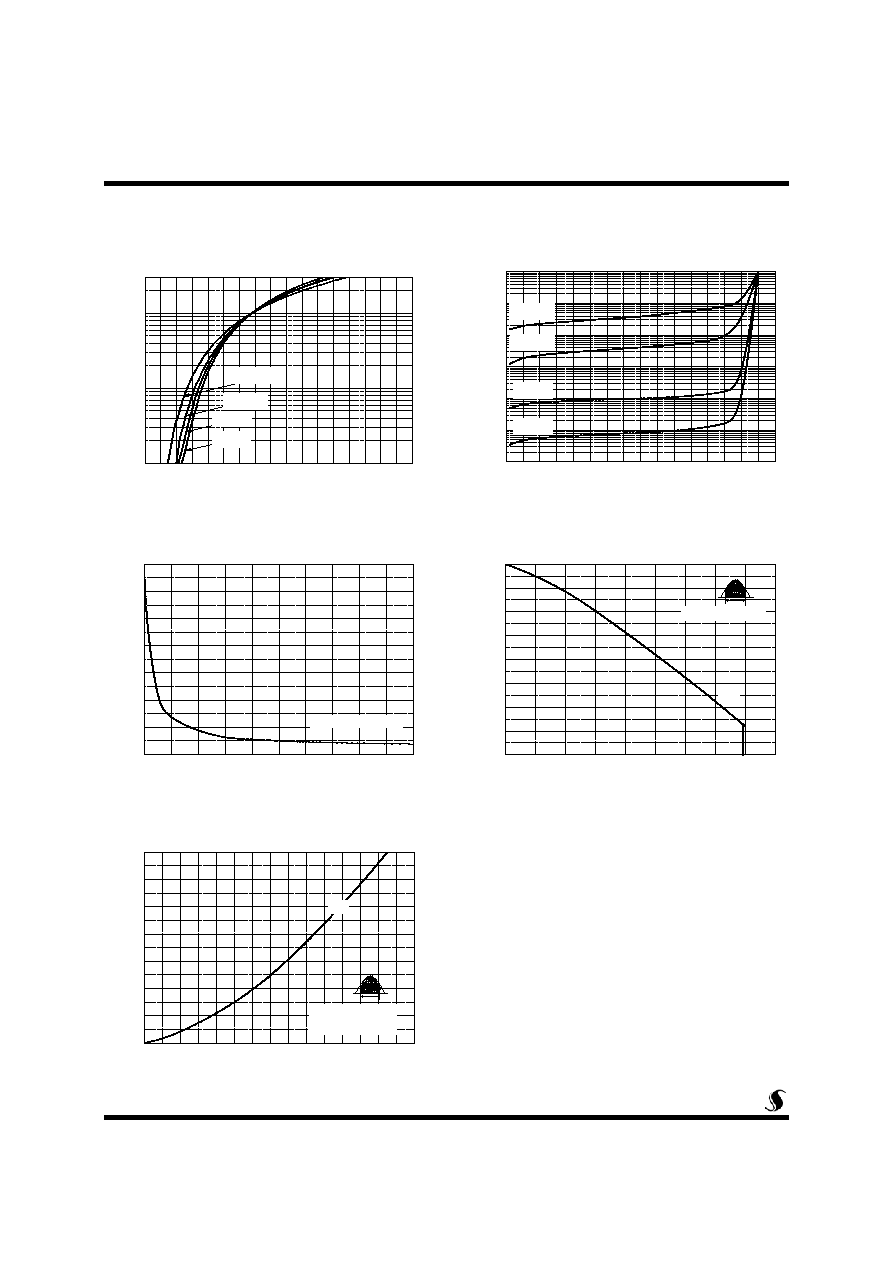0.0
0.5
1.0
1.5
2.0
2.5
3.0
3.5
4.0
1
10
100
T
C
=150
o
C
T
C
=100
o
C
T
C
=50
o
C
I
FA
V
,
F
o
rward current
[
A
]
V
F
, Forward voltage drop[V]
T
C
=25
o
C
300
0
100
200
300
400
500
600
700
800
10
0
10
1
10
2
10
3
10
4
10
5
10
6
I
R
,
Le
ak
age

c
u
rren
t
[uA]
V
R
, Reverse voltage[V]
T
C
=150
o
C
T
C
=100
o
C
T
C
=50
o
C
T
C
=25
o
C
0
20
40
60
80
100
120
140
0
50
100
150
200
250
300
350
180
o
P
D
,
Ma
xi
mu
m Pow
e
r
D
i
ss
i
p
a
t
i
o
n[W
]
I
FAV
, Forward current[A]
Conduction Angle
T
J
=150
o
C
0
20
40
60
80
70
80
90
100
110
120
130
140
150
T
C
,
Ma
xi
m
u
m

A
l
lo
wabl
e Cas
e

T
e
m
p
er
a
t
ur
e
[
o
C]
I
FAV
, Forward current[A]
Rthjc = 0.47
o
C/W
180
o
0
20
40
60
80
100
120
130
140
150
160
170
180
190
C
T
,
J
u
nc
t
i
o
n

Ca
pa
c
i
t
a
n
c
e[
pF
]
V
R
, Reverse voltage[V]
Freuqency 1[MHz]
SDW80S600
4/5
Fig 7. Forward average current vs.
forward voltage drop
Fig 8. Reverse leakage current vs.
reverse voltage
Fig 9. Juntion capacitance vs. reverse
voltage.
Fig 10. Maximum allowable case
temperature
Fig 11. Maximum power dissipation vs.
forward average current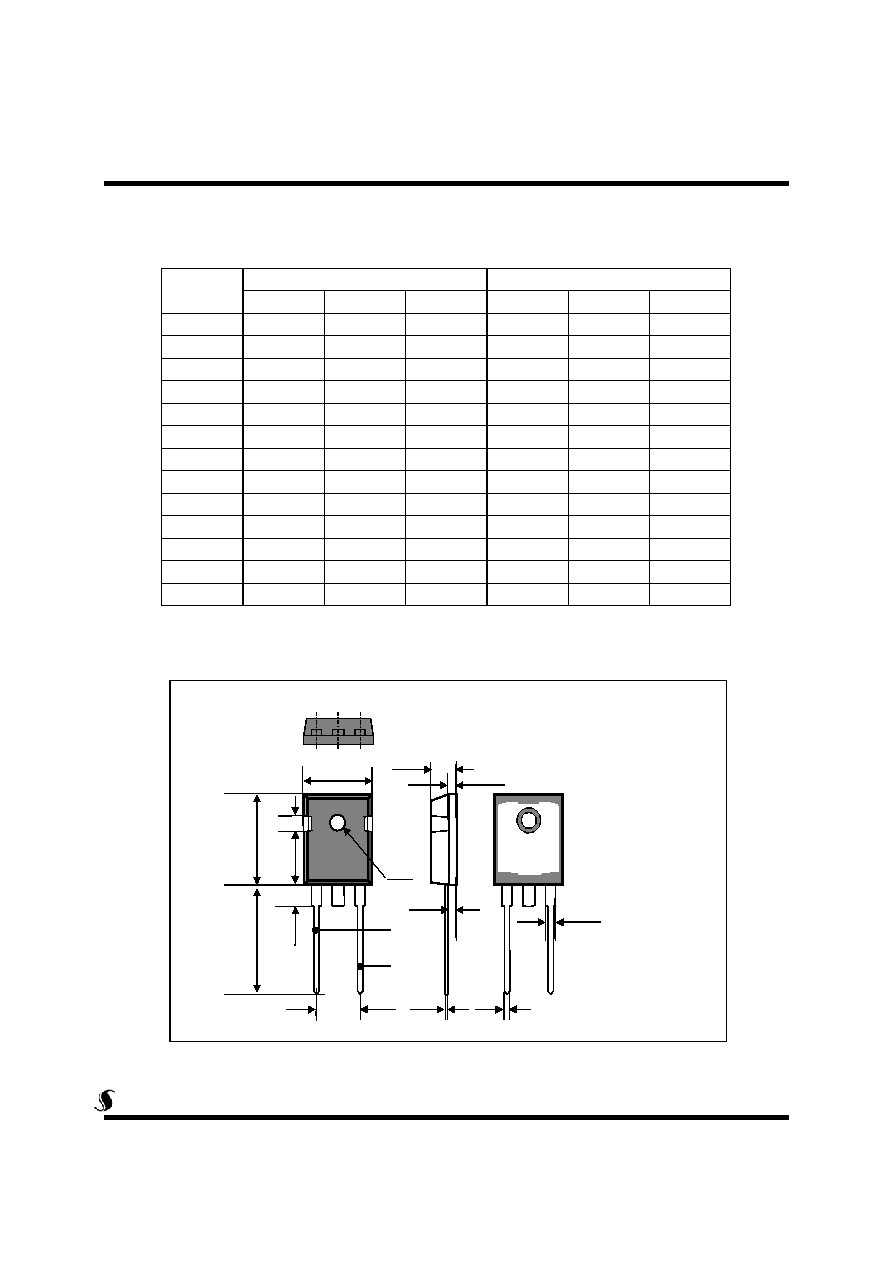Dim.
mm
Inch
Min.
Typ.
Max.
Min.
Typ.
Max.
A
15.77
16.03
0.621
0.631
B
20.80
21.10
0.819
0.831
C
20.05
20.31
0.789
0.800
D
4.48
4.58
0.176
0.180
E
4.27
4.37
0.168
0.172
F
10.64
11.16
0.418
0.440
G
4.90
5.16
0.193
0.203
H
1.90
2.06
0.075
0.081
I
2.35
2.45
0.093
0.096
J
0.6
0.024
K
1.93
2.13
0.076
0.084
L
1.07
1.33
0.042
0.052
3.56
3.66
0.140
0.144
TO-247 AD Package Dimension
SDW80S600
5/5
1. Cathode
2. Anode
A
B
C
E
I
1
D
2
G
H
L
K
J
F© 2019 • ICSheet• Contact form• Main page